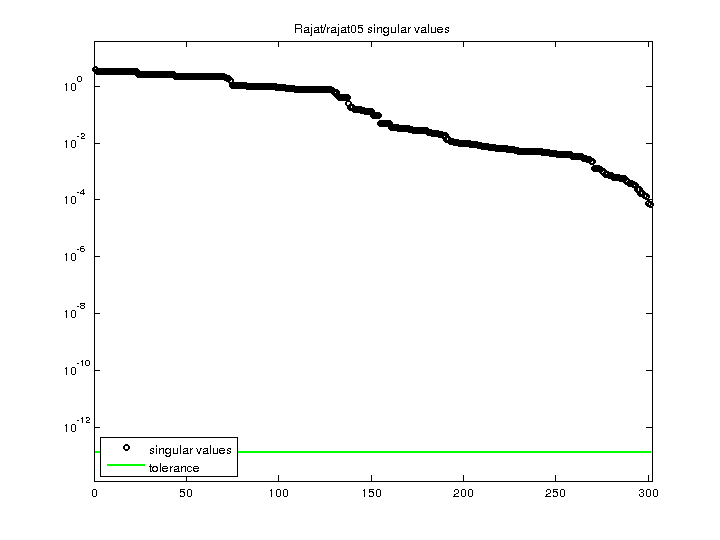Matrix: Rajat/rajat05

Description: Rajat/rajat05 circuit simulation matrix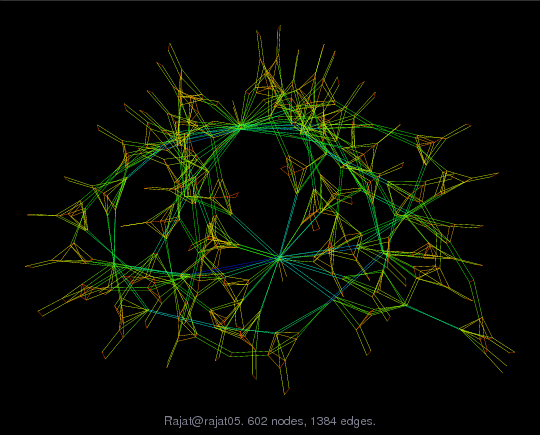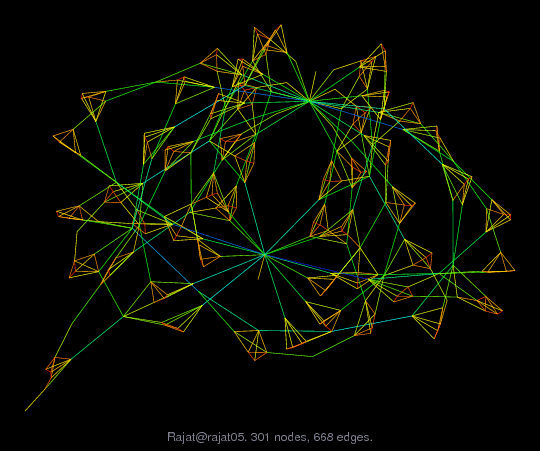(bipartite graph drawing) (graph drawing of A+A')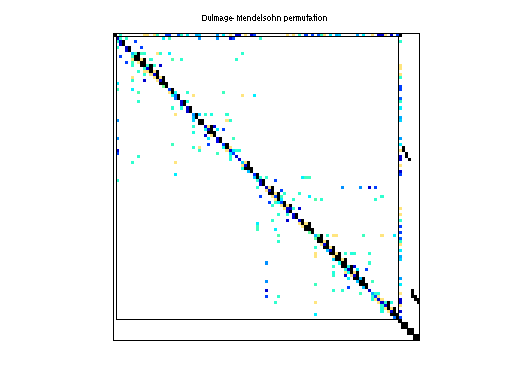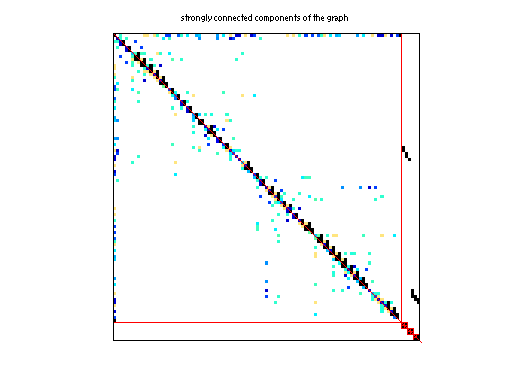• Matrix group: Rajat
• download as a MATLAB mat-file, file size: 6 KB. Use UFget(1189) or UFget('Rajat/rajat05') in MATLAB.

 Matrix properties number of rows 301 number of columns 301 nonzeros 1,250 structural full rank? yes structural rank 301 # of blocks from dmperm 15 # strongly connected comp. 9 explicit zero entries 134 nonzero pattern symmetry 77% numeric value symmetry 71% type real structure unsymmetric Cholesky candidate? no positive definite? no

 author Rajat editor T. Davis date 2004 kind circuit simulation problem 2D/3D problem? no

 Ordering statistics: result nnz(chol(P*(A+A'+s*I)*P')) with AMD 1,169 Cholesky flop count 4.7e+03 nnz(L+U), no partial pivoting, with AMD 2,037 nnz(V) for QR, upper bound nnz(L) for LU, with COLAMD 1,938 nnz(R) for QR, upper bound nnz(U) for LU, with COLAMD 3,587

Note that all matrix statistics (except nonzero pattern symmetry) exclude the 134 explicit zero entries.

 SVD-based statistics: norm(A) 3.83917 min(svd(A)) 7.11913e-05 cond(A) 53927.5 rank(A) 301 sprank(A)-rank(A) 0 null space dimension 0 full numerical rank? yes

 singular values (MAT file): click here SVD method used: s = svd (full (A)) ; status: ok